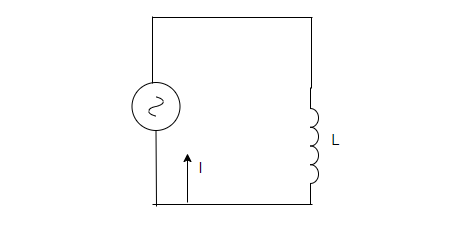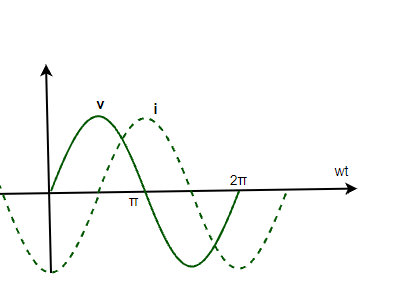GFG App
Open AppBrowser
Continue

# AC Voltage Applied to an Inductor

Alternating Currents and Voltages vary and change their directions with time. They are widely used in modern-day devices and electrical systems because of their numerous advantages. Circuits in everyday life consist of resistances, capacitors, and inductances. Inductors are devices that store energy in their magnetic fields when current flows through them. These devices are found in a lot of applications so it is essential to understand the behavior of the circuit when capacitance is connected to a voltage source. Let’s look at these concepts in detail.

### AC Voltage Applied to an Inductor

The figure given below shows an ac circuit. Here, an ac voltage source is connected to a capacitor. The expression for the voltage from the voltage source is given by v = vmsin(ωt). An inductor is a passive electrical device that stores energy when the magnetic field is created inside due to the flowing electric current. Inductor either acquires or losses charge. The effect of the inductor is called inductance. An inductor when connected to a voltage source draws current from the source so as to charge itself.

The voltage across an inductor is measured by the change in electric current through the inductor.In the circuit given above, an inductor is connected to an alternating voltage source denoted by ~.  Assuming that the resistance in the windings is negligible. As the current changes, the inductor acquires potential. The circuit is a purely inductive circuit.

Using the kirchhoff’s rule,The second term in the equation is the self-induced emf inside the inductor. Let the inductance be denoted by “L”.This is an equation in terms of current. It is expressed in terms of derivatives. The slope of the current is varying with time. To obtain the value of the current, the equation must be integrated.⇒The integral constant has dimensions of current and is time-independent. Since the emf voltage source oscillates symmetrically around zero, the current in the circuit also oscillates so that no time independent exists. Thus, the value of the constant is zero.Rearranging the above equation,

i = imsin(ωt – π/2)

Here, im = vm/ωL. It is the amplitude of the oscillating current. It can also be re-written as,This equation when compared to ohm’s law gives ωL as resistance. It is called inductive reactance and it is denoted by XL

Now, the amplitude of the current becomes,

imThe dimensions of inductive reactance are the same as resistance and its SI unit is Ohms. Intuitively speaking, inductive reactance limits the current of a purely inductive circuit in the same way as resistance limits the current in a usual resistive circuit.

Previous equations show that the current is behind the voltage in terms of phase. There is a phase difference of. The figure given below shows the variation of voltage and current with time.The power dissipated in a purely inductive circuit can be derived using the instantaneous equation of power,

Pc = iv

⇒ Pc = (imsin(ωt – π/2))(vmsin(ωt))

⇒ Pc = -imvmcos(ωt)sin(ωt)

⇒ Pc = – imvm/2sin(2ωt)

The average power dissipated in this case,

Pav = 0

### Sample Problems

Question 1: A inductor of 12mH is connected to a voltage source of frequency 50Hz. Find the reactance of the inductance.

The reactance of the inductance is given by,

XL = ωL

Given:

f = 50Hz

L = 12 mH

ω = 2πf

⇒ ω = 2π(50)

⇒ ω = 100π

Plugging the values in the equation,

X = ωL

⇒ XL = 100π × 12 × 10-3

⇒ XL = 1200π × 10-3

⇒ XL = 37.7 × 10-3

⇒ XL = .0377 Ohms

Question 2: A inductor of 24mH is connected to a voltage source of frequency 50Hz. Find the reactance of the inductance.

The reactance of the inductance is given by,

X = ωL

Given:

f = 50Hz

L = 24 mH

ω = 2πf

⇒ ω = 2π(50)

⇒ ω = 100π

Plugging the values in the equation,

XL = ωL

⇒ XL = 100π × 24 × 10-3

⇒ XL = 2400π × 10-3

⇒ XL = 75.4 × 10-3

⇒ XL = .0754 Ohms

Question 3: A capacitor of 1 mH is connected to a voltage source given by,

v = 50sin(20t)

Find the amplitude of the current.

The reactance of the capacitance is given by,

X = ωL

Given:

ω = 20

L = 1 mF

Plugging the values in the equation,

X = ωL

⇒ XL = 0.02

Amplitude of the current will be,

im = vm/Xc

⇒ im = 50 / (0.02)

⇒ im = 5000A

Question 4: A sinusoidally varying current is applied to an inductive circuit. The impedance of the inductance is given as 2 ohms. Find the power dissipated in the circuit if the voltage source has an RMS voltage of 45V.

The Average power is given by,

P = VIcos(φ)

Since the circuit is purely inductive circuit. The, phase angle will be 90°.

cos(φ) = 0

plugging the values in the equation,

P = VIcos(φ)

P = 0.

Question 5: A capacitor of 10mH is connected to a voltage source given by,

v = 50sin(20t)

Find the amplitude of the current.

The reactance of the capacitance is given by,

X = ωL

Given:

ω = 20

L = 10mH

Plugging the values in the equation,

XL = ωL

⇒ XL = 20 × 10 ×10-3

⇒ XC = 0.2

Amplitude of the current will be,

im = vm/Xc

⇒ im = 50 / (0.2)

⇒ im = 100A

My Personal Notes arrow_drop_up